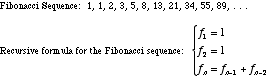index: click on a letter A B C D E F G H I J K L M N O P Q R S T U V W X Y Z A to Z index index: subject areas numbers & symbols sets, logic, proofs geometry algebra trigonometry advanced algebra & pre-calculus calculus advanced topics probability & statistics real world applications multimedia entrieswww.mathwords.com about mathwords website feedback

 Fibonacci Sequence The sequence of numbers 1, 1, 2, 3, 5, 8, 13, 21, 34, . . . for which the next term is found by adding the previous two terms. This sequence is encountered in many settings, from population models to botany. Note: The sequence of ratios of consecutive terms has the Golden Mean as its limit.